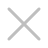• 巧夺天工
• 中信红木
• 红古轩
• 卓木王
• 泰和园
• 明堂红木
• 古森红木
• 国寿红木
• 居典红木
• 国方家居
• 万事红
• 华行红木
• 雅宋红木
• 国祥红木
• 大家之家
• 御乾堂
• 凹凸传奇
• 闲适红木
• 东遇家居
• 清韵红木
• 喜迎红红木
• 素创
• 微凹传奇
• 老周家居
• 华厦·大不同
• 本木上造
• 东方之信红木
• 波记家具
• 顺泰轩
• 华夏一品
• 苏阳红
• 宝鹰红木
• 雅典红木
• 志成红木
• 美联红木
• 慕东方红木
• 名雅居红木(耐匠红木)
• 名匠艺林
• 拾贰空间
• 沽之大匠
• 大门户
• 东邦红木
• 祥瑞坊红木
• 森木家具
• 大成尚品
• 鼎顺红木
• 永华红木
• 一品居、宏达豪典
• 鸿运堂
• 0-10000
• 10001-20000
• 20001-40000
• 40001-80000
• 80000以上
• 面议
• 大果紫檀(缅甸花梨)
• 阔叶黄檀(印尼黑酸枝)
• 刺猬紫檀
• 交趾黄檀(大红酸枝)
• 巴里黄檀(花枝)
• 降香黄檀(海南黄花梨)
• 檀香紫檀(小叶紫檀)
• 奥氏黄檀(白酸枝)
• 微凹黄檀(红酸枝)
• 红酸枝
• 东非黑黄檀(紫光檀)
• 卢氏黑黄檀(大叶紫檀)
• 越南黄花梨
• 染料紫檀(非洲血檀)
• 鸡翅木
• 古夷苏木(巴花)
• 红铁木豆(小叶红檀)
• 螺穗木(非洲檀香)
• 沉贵宝(东非酸枝)
• 红贵宝(非洲酸枝)
• 小巴花(东非香花梨)
• 柬埔寨黑酸枝
• 马岛黑酸枝
• 条纹乌木
• 安哥拉紫檀(红高棉)
• 非洲紫檀(红花梨)
• 其他名贵硬木
• 其他工艺品
• 其他
• 书房系列
• 客厅系列
• 餐厅系列
• 茶室系列
• 套房系列
• 其它系列
• 新古典
• 新中式
• 明清古典
• 欧式古典
• 其他风格
• 香几类
• 屏风类
• 柜架类
• 宝座类
• 凳墩类
• 坐椅类
• 床榻类
• 桌案类
• 沙发类

• 巧夺天工
• 中信红木
• 红古轩
• 明堂红木
• 卓木王
• 古森红木
• 泰和园
• 国寿红木
• 居典红木
• 万事红
• 国方家居
• 东遇家居
• 华行红木
• 凹凸传奇
• 国祥红木
• 大家之家
• 雅宋红木
• 御乾堂
• 喜迎红红木
• 闲适红木
• 苏阳红
• 波记家具
• 老周家居
• 拾贰空间
• 东方之信红木
• 雅典红木
• 本木上造
• 美联红木
• 华厦·大不同
• 东邦红木
• 华夏一品
• 名雅居红木(耐匠红木)
• 鼎顺红木
• 志成红木
• 森木家具
• 鸿运堂
• 清韵红木
• 顺泰轩
• 一品居、宏达豪典
• 永华红木
• 宝鹰红木
• 祥瑞坊红木
• 沽之大匠
• 名匠艺林
• 微凹传奇
• 大成尚品
• 大门户
• 素创
• 慕东方红木

• 0-10000
• 10001-20000
• 20001-40000
• 40001-80000
• 80000以上
• 面议

• 大果紫檀(缅甸花梨)
• 阔叶黄檀(印尼黑酸枝)
• 刺猬紫檀
• 交趾黄檀(大红酸枝)
• 巴里黄檀(花枝)
• 降香黄檀(海南黄花梨)
• 檀香紫檀(小叶紫檀)
• 奥氏黄檀(白酸枝)
• 微凹黄檀(红酸枝)
• 红酸枝
• 东非黑黄檀(紫光檀)
• 卢氏黑黄檀(大叶紫檀)
• 越南黄花梨
• 染料紫檀(非洲血檀)
• 鸡翅木
• 古夷苏木(巴花)
• 红铁木豆(小叶红檀)
• 螺穗木(非洲檀香)
• 沉贵宝(东非酸枝)
• 红贵宝(非洲酸枝)
• 小巴花(东非香花梨)
• 柬埔寨黑酸枝
• 马岛黑酸枝
• 条纹乌木
• 安哥拉紫檀(红高棉)
• 非洲紫檀(红花梨)
• 其他名贵硬木
• 其他工艺品
• 其他

• 书房系列
• 客厅系列
• 餐厅系列
• 茶室系列
• 套房系列
• 其它系列

• 新古典
• 新中式
• 明清古典
• 欧式古典
• 其他风格

• 香几类
• 屏风类
• 柜架类
• 宝座类
• 凳墩类
• 坐椅类
• 床榻类
• 桌案类
• 沙发类